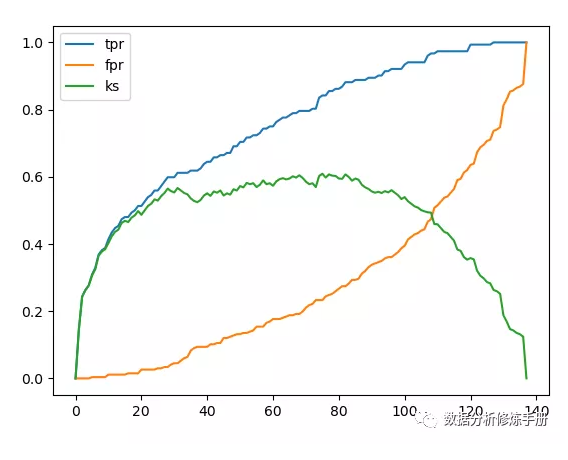# 【模型评估_指标】

## 一、回归模型评估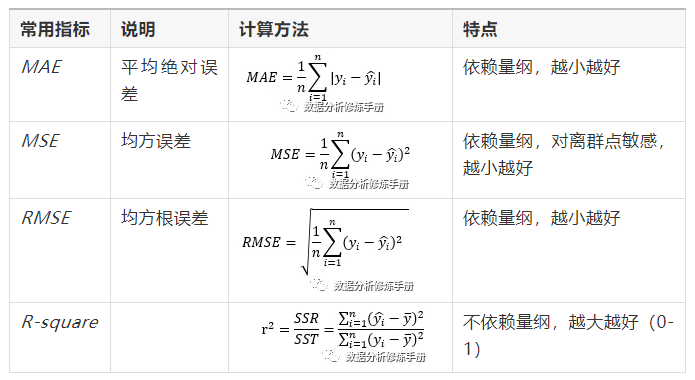``````##建立回归模型，预测y_test
from sklearn.linear_model import LinearRegression
lr = LinearRegression()
lr.fit(x_train,y_train)
y_pred = lr.predict(x_test)## MAE评估
from sklearn.metrics import mean_absolute_error
mean_absolute_error(y_test,y_pred)## MSE评估
from sklearn.metrics import mean_squared_error
mean_squared_error(y_test,y_pred)## R-square评估
from sklearn.metrics import r2_score
r2_score(y_test,y_pred)
``````

## 二、分类模型评估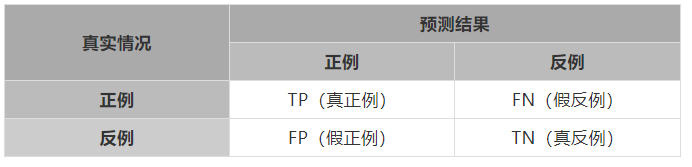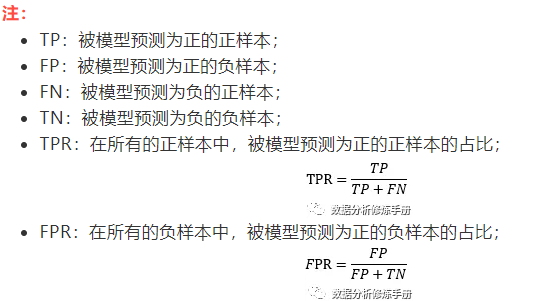``````## 建立模型（这里我们用tatanic做数据源，选用随机森林模型）
from sklearn.ensemble import RandomForestClassifier
rf = RandomForestClassifier(random_state=1)
rf.fit(x_train, y_train)
## 测试集结果预测
y_pred_prob = rf.predict_proba(x_test)  ## 返回预测各个类别的概率，第一列返回0的概率，第二列返回1的概率，两概率之和为1
y_pred = rf.predict(x_test)  ## 返回预测值（默认阈值为0.5），y_pred[:,1]>0.5返回1，否则返回0########## 混淆矩阵
## 混淆矩阵
from sklearn.metrics import confusion_matrix
from sklearn.metrics import plot_confusion_matrix
confusion_matrix(y_test,y_pred)
import matplotlib.pyplot as plt
## 绘制混淆矩阵
plot_confusion_matrix(rf, x_test, y_test)
plt.show()
``````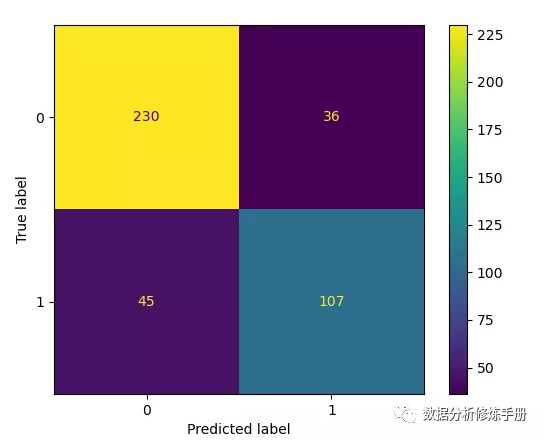## ****** 准确率（Accuracy） ******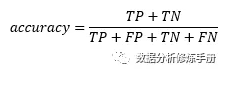• 当样本不均衡时不建议使用
``````########## 准确率
from sklearn.metrics import accuracy_score
accuracy_score(y_test,y_pred)   ## 0.80622009569378
``````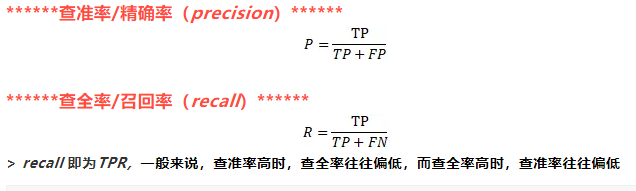``````
########## 查准率、查全率
## 查准率/精确率
from sklearn.metrics import precision_score
precision_score(y_test,y_pred)  ## 0.7482517482517482## 查全率/召回率
from sklearn.metrics import recall_score
recall_score(y_test,y_pred)   ## 0.7039473684210527## 汇总数据
from sklearn.metrics import precision_recall_fscore_support
precision_recall_fscore_support(y_test, y_pred)``````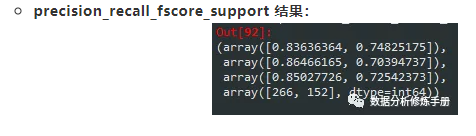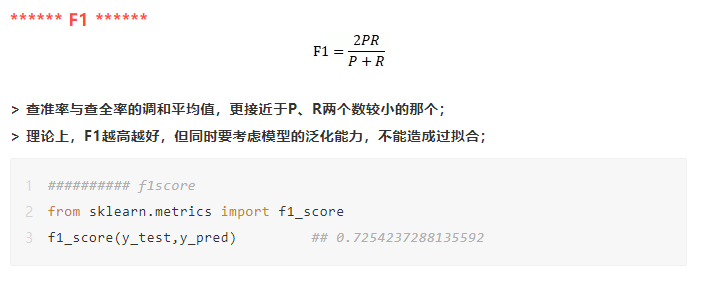## ****** P-R图 ******

• 横轴：recall，纵轴：precision（一个好的模型应该尽可能接近图形的右上角）
• 设置不同的阈值，计算出相应的precision与recall，然后绘制出如下所示的图形，如果一条曲线c被另外一条曲线b完全包围，则曲线b的性能优于曲线c，如果不能直接判断哪个曲线的性能更好，可以根据平衡点(BEP)，即P=R时，哪条曲线的precision更高，则性能更好；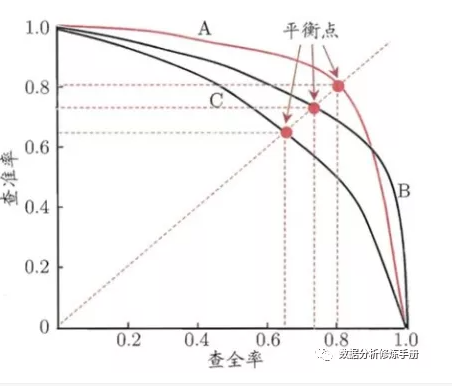``````
## P-R图（两种方式实现）
# 法1
from sklearn.metrics import precision_recall_curve
precision, recall, thresholds = precision_recall_curve(y_test, y_pred_prob[:,1])
plt.plot(recall,precision)
# 法2
from sklearn.metrics import plot_precision_recall_curve
plot_precision_recall_curve(rf,x_test,y_test)
``````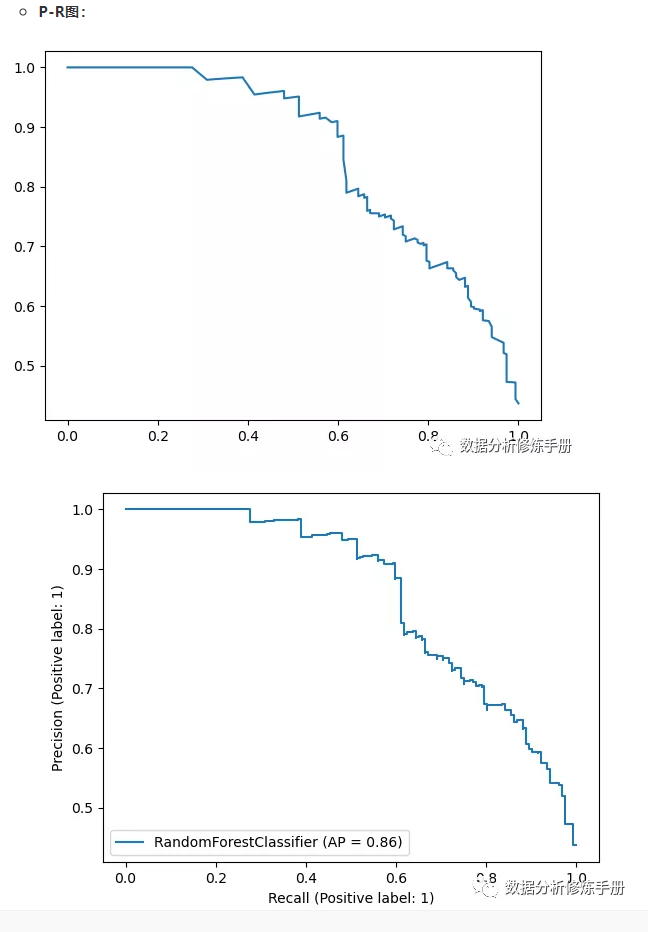## ****** ROC/AUC ******

• 横轴：FPR，纵轴：TPR（一个好的模型应该尽可能接近图形的左上角）

• 与P-R图相似，同样是根据设置不同的阈值，来画出图形，不同的是P-R使用的precision，而ROC使用的是FPR，由此可以看出，P-R图只关心正例的情况，因此，在类别不平衡问题中PR曲线被广泛认为优于ROC曲线；

TPR=0、FPR=0：把每个样本都预测为负类的模型

TPR=1、FPR=1：把每个样本都预测为正类的模型

TPR=1、FPR=0：理想模型

• 当测试集中的正负样本的分布变化的时候，ROC曲线能够保持不变。因为TPR聚焦于正例，FPR聚焦于负例，使其成为一个比较均衡的评估方法

• AUC值为ROC曲线所覆盖的区域面积，AUC越大，模型效果越好，通常0.5<AUC<1，>=0.75时建立的评分卡可靠

``````
########## roc/auc
from sklearn.metrics import roc_auc_score
roc_auc_score(y_test, y_pred_prob[:,1])  ## 0.8952067669172933from sklearn.metrics import roc_curve
from sklearn.metrics import auc
fpr, tpr, thresholds = roc_curve(y_test, y_pred_prob[:,1])
auc(fpr, tpr) ## 0.8952067669172933
plt.plot(fpr, tpr)from sklearn.metrics import plot_roc_curve
plot_roc_curve(rf, x_test, y_test)
``````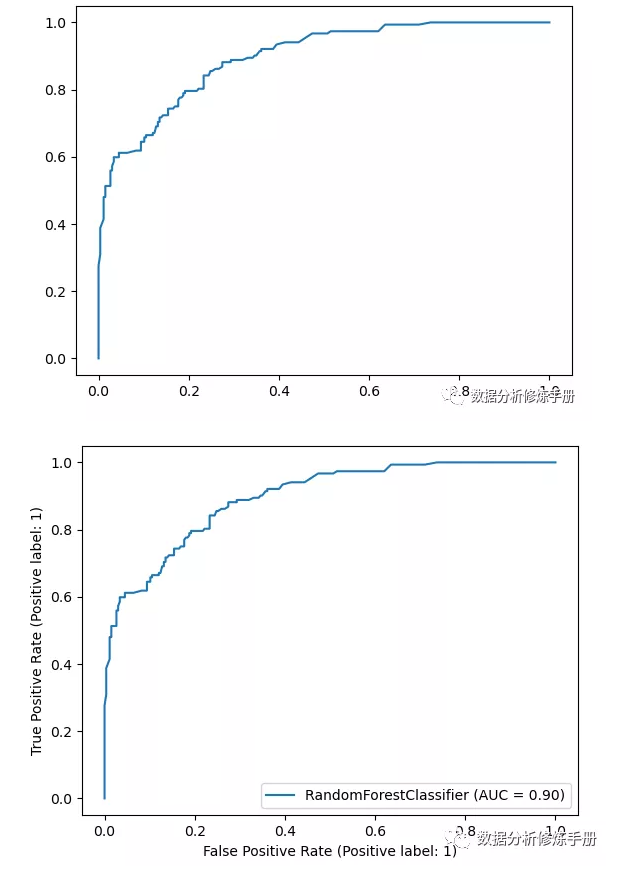## ****** KS ******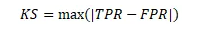• 取值范围：0-1之间；
• 指标衡量的是好坏样本累计分布之间的差值，好坏样本累计差异越大，KS指标越大，模型的风险区分能力越强；
• KS的计算步骤如下：
1. 计算每个评分区间的好坏账户数